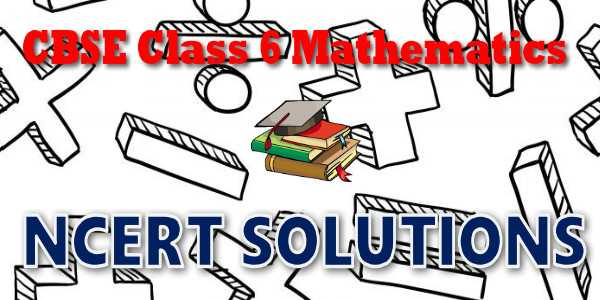# CBSE - Class 06 - Mathematics - Mensuration - NCERT Solutions## CBSE, JEE, NEET, NDA

Question Bank, Mock Tests, Exam Papers

NCERT Solutions, Sample Papers, Notes, Videos

## NCERT Solutions for Mathematics Mensuration## myCBSEguide App

Complete Guide for CBSE Students

NCERT Solutions, NCERT Exemplars, Revison Notes, Free Videos, CBSE Papers, MCQ Tests & more.

# NCERT solutions for class 6  Mathematics Mensuration## NCERT Class 6 Mathematics Chapter-wise Solutions

Maths Part I

• Chapter 1 - Knowing Our Numbers
• Chapter 2 - Whole Numbers
• Chapter 3 - Playing with Numbers
• Chapter 4 - Basic Geometrical Ideas
• Chapter 5 - Understanding Elementary Shapes
• Chapter 6 - Integers
• Chapter 7 - Fractions
• Chapter 8 - Decimals
• Chapter 9 - Data Handling
• Chapter 10 - Mensuration
• Chapter 11 - Algebra
• Chapter 12 - Ratio and Proportion
• Chapter 13 - Symmetry
• Chapter 14 - Practical Geometry

## CBSE class 6th Mathematics have one books. Book has chapters and topics.

• NCERT Mathematics Book Class 6

Here is the list of topics covered under each chapter of class 6 Mathematics NCERT text book.

• Exercise 1.1
• Exercise 1.2
• Exercise 1.3

• Exercise 2.1
• Exercise 2.2
• Exercise 2.3

• Exercise 3.1
• Exercise 3.2
• Exercise 3.3
• Exercise 3.4
• Exercise 3.5
• Exercise 3.6
• Exercise 3.7

• Exercise 4.1
• Exercise 4.2
• Exercise 4.3
• Exercise 4.4
• Exercise 4.5
• Exercise 4.6

• Exercise 5.1
• Exercise 5.2
• Exercise 5.3
• Exercise 5.4
• Exercise 5.5
• Exercise 5.6
• Exercise 5.7
• Exercise 5.8
• Exercise 5.9

• Exercise 6.1
• Exercise 6.2
• Exercise 6.3

• Exercise 7.1
• Exercise 7.2
• Exercise 7.3
• Exercise 7.4
• Exercise 7.5
• Exercise 7.6

• Exercise 8.1
• Exercise 8.2
• Exercise 8.3
• Exercise 8.4
• Exercise 8.5
• Exercise 8.6

• Exercise 9.1
• Exercise 9.2
• Exercise 9.3
• Exercise 9.4

### 10. Mensuration

• Exercise 10.1
• Exercise 10.2
• Exercise 10.3

### 11. Algebra

• Exercise 11.1
• Exercise 11.2
• Exercise 11.3
• Exercise 11.4
• Exercise 11.5

### 12. Ratio and Proportion

• Exercise 12.1
• Exercise 12.2
• Exercise 12.3

### 13. Symmetry

• Exercise 13.1
• Exercise 13.2
• Exercise 13.3

### 14. Practical Geometry

• Exercise 14.1
• Exercise 14.2
• Exercise 14.3
• Exercise 14.4
• Exercise 14.5
• Exercise 14.6

## NCERT Solutions Chapter 10: Mensuration

When we talk about some plane figures as shown below we think of their regions and their boundaries. We need some measures to compare them. We look into these now.

You can make them with a wire or a string. If you start from the point S in each case and move along the line segments then you again reach the point S. You have made a complete round of the The distance covered is equal to the length of wire used to draw the figure. This distance is known as the perimeter of the closed figure. It is the length of the wire needed to form the figures. The idea of perimeter is widely used in our daily life.

• A farmer who wants to fence his field.
• An engineer who plans to build a compound wall on all sides of a house.
• A person preparing a track to conduct sports.

All these people use the idea of ‘perimeter’. Give five examples of situations where you need to know the perimeter. Perimeter is the distance covered along the boundary forming a closed figure when you go round the figure once.

## NCERT Solutions for Class 6th Mathematics

NCERT Solutions Class 6 Mathematics PDF (Download) Free from myCBSEguide app and myCBSEguide website. Ncert solution class 6 Mathematics includes text book solutions from  NCERT Solutions for CBSE Class 6 Mathematics have total 14 chapters. Class 6 Mathematics ncert Solutions in pdf for free Download are given in this website. Ncert Mathematics class 6 solutions PDF and Mathematics ncert class 6 PDF solutions with latest modifications and as per the latest CBSE syllabus are only available in myCBSEguide.

## NCERT Solutions for Class 5

NCERT Solutions for Class 4

## NCERT Solutions for Class 3## myCBSEguide

Trusted by 1 Crore+ Students

#### CBSE Test Generator

Create papers in minutes

Print with your name & Logo

3 Lakhs+ Questions

Solutions Included

Based on CBSE Blueprint

Best fit for Schools & Tutors

#### Work from Home

• Work from home with us
• Create questions or review them from home

No software required, no contract to sign. Simply apply as teacher, take eligibility test and start working with us. Required desktop or laptop with internet connection